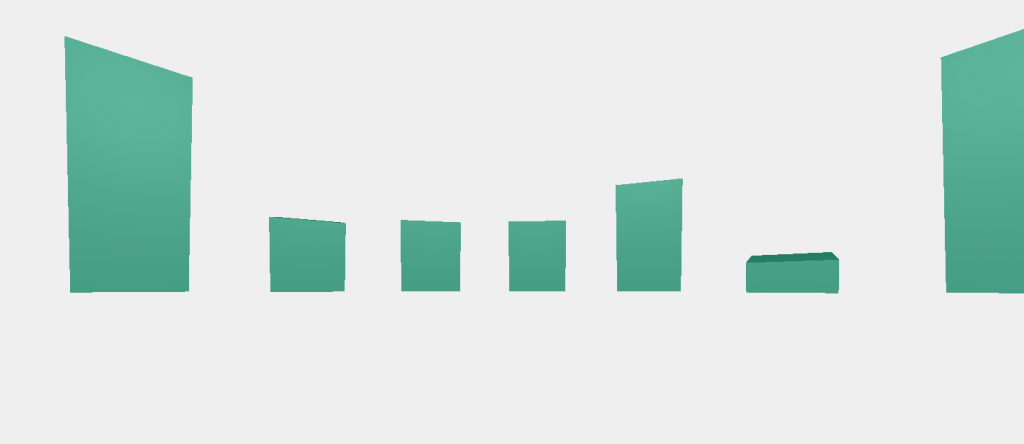#2017 iT 邦幫忙鐵人賽
DAY 29
0
Modern Web

## [Day29] Aframe 範例 - 3D 與 d3.js

demo

### 基本環境設置

``````<a-scene>
<a-light color="#27cc95" position="1 0 0" type="ambient"></a-light>
<a-entity light="type: point; color: #fff; intensity:0.5" position="0 5 0"></a-entity>
<a-entity position="0 0 0" rotation="0 0 0">
<a-entity camera look-controls wasd-controls>
</a-entity>
</a-entity>
</a-scene>
``````

• 設定 canvas renderer
• 決定 lilght camera 等位置
• 設定 webVR
• `scene` 裡頭加入 UI

A scene is represented by the `<a-scene>` element. The scene is the global root object, and all entities are contained within the scene.

• point
• ambient
• directional

### 加入資料

d3 的部分，這邊就不多說明了。

``````var data = [
120, 10, 13, 45, 67,
54, 12, 22, 200, 120,
123, 154, 243, 100, 40,
44, 45, 65, 16, 94, 33
];

var hscale = d3.scale
.linear()
.domain([0, d3.max(data)])
.range([0,10])

var scene = d3.select("a-scene");

var cubes = scene
.selectAll("a-cube.bar")
.data(data)
.enter()
.append("a-cube")
.classed("bar", true)
.attr({
position: function(d,i) {
var theta = (i/data.length) * (2 * Math.PI)
var x = radius * Math.cos(theta);
var y = hscale(d)/2;
var z = radius * Math.sin(theta);
return x + " " + y + " " + z
},
rotation: function(d,i) {
var x = 0;
var y = -360 * i / data.length;
var z = 10;
return x + " " + y + " " + z
},
height: function(d,i) {
return hscale(d)
}
});
``````

aframe 會自動將我們所寫的 tag 全部轉為 canvas，這樣子我們就可以輕易地用 HTML tag 來做開發，也比較方便 debug。